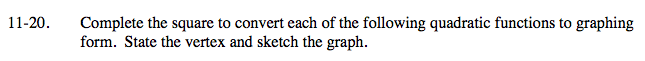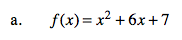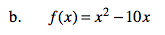Home > A2C > Chapter 11 > Lesson 11.1.2 > Problem11-20

11-20.
1. Complete the square to convert each of the following quadratic functions to graphing form. State the vertex and sketch the graph. Homework Help ✎

1. f(x) = x2 + 6x + 7

2. f(x) = x2 − 10xf(x) = (x2 + 6x + 9) − 9 + 7

f(x) = (x + 3)2 − 2

vertex: (−3, −2)See part (a).